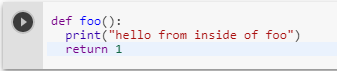2 views
in Python

What is the simple basic explanation of what the return statement is, how to use it in Python?

And what is the difference between it and the print statement?

by (106k points)
edited by

The purpose of the return statement in any language causes your function to exit and hand back value to its caller. The point of functions, in general, is to take in inputs and return something. The return statement is used when a function is ready to return a value to its caller.

Example:-

def foo():

print("hello from inside of foo")

return 1You can see return has ended the function.

by (20.3k points)

In python, you can define a function with "def" and generally, and end the function with "return". A function of variable x can be denoted as f(x).

You must be wondering what this function exactly does? Assume, this function adds 2 to x. Hence, it'll be like f(x)=x+2

Now, the code of this function will be like:

def A_function (x):

return x + 2

After defining the function, you can try using that for any variable and get result. Such as:

print A_function (2)

>>> 4

You can just write the code slightly differently, such as:

def A_function (x):

y = x + 2

return y

print A_function (2)

The output will be "4".

Now, you can even try using the code given below:

def A_function (x):

x = x + 2

return x

print A_function (2)

That would also give 4. See, that the "x" beside return actually means (x+2), not x of "A_function(x)". I believe from this example, you can understand why do we use return command.